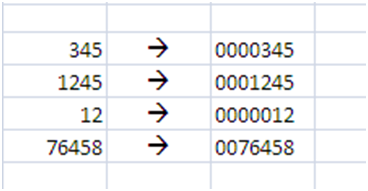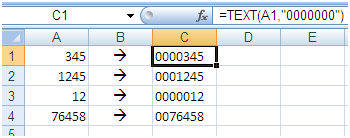# How to Add Zero’s before any Figure in Excel

### Some time it is required to add Zero’s before any number to make it of constant length e.g.### In Excel it can be done by using simple formula of Text for example

If you want to convert a number to 7 Digit number by adding Zero before it can be don’t this by this formula

If figures are written in coulomb A then past this formula in next coulomb

=text(A1,”0000000″)Add number of zeros between inverted comas that much Digits figure you want to get

If you want to convert 123 à 00123 the its 5 digits figure so formula will become

=text(A1,”00000″)